Get instant live expert help with Excel or Google Sheets“My Excelchat expert helped me in less than 20 minutes, saving me what would have been 5 hours of work!”

#### Post your problem and you’ll get Expert help in seconds.

Your message must be at least 40 characters
Our professional Expert are available now. Your privacy is guaranteed.

# How to insert subtotals in Excel

Inserting subtotals can be done manually, where we insert a subtotal for each group of items in our list.  We can also use the Excel SUBTOTAL function, which offers several functions to be used to insert subtotals.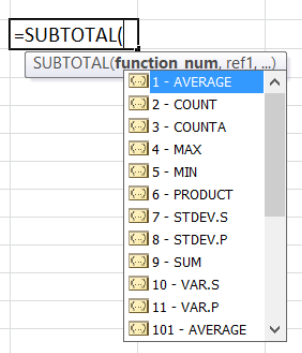Figure 1. Excel SUBTOTAL function

However, this task could become tedious when handling large data sets.  Fortunately, Excel has a tool for subtotals that makes inserting subtotals in Excel so much easier.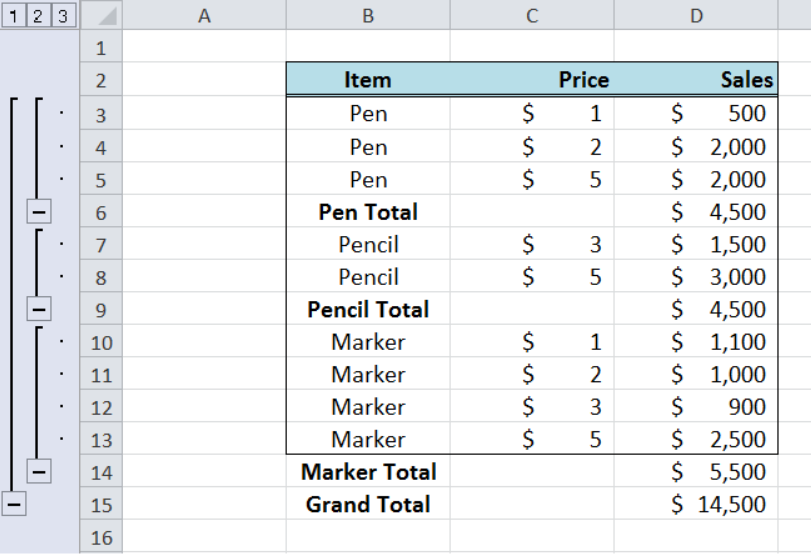Figure 2.  Final result:  How to insert subtotals in Excel

The image above shows that we are able to summarize data in a range of data by inserting subtotals.  This article shows how to insert subtotals in Excel for versions 2016, 2013 or lower.

## How to insert subtotals in excel?

Suppose we want to insert a subtotal for the sum of sales for each group of items in column B:  Pen, Pencil and Marker. We follow these steps:

Step 1.  Select the whole list/database B2:D11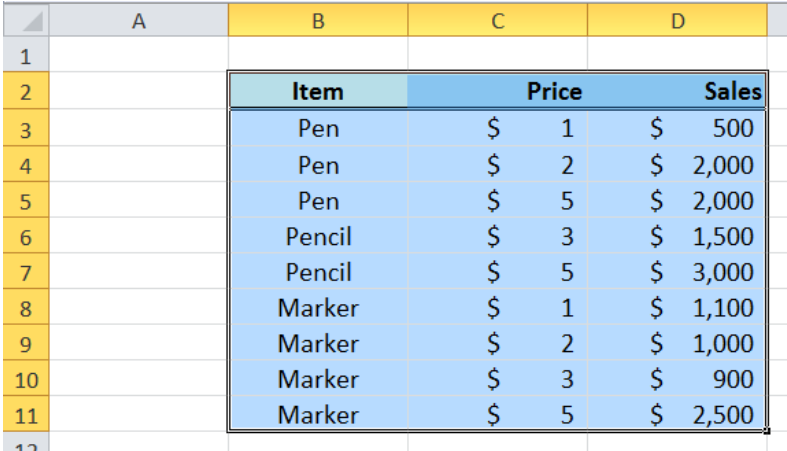Figure 3.  Sample data to insert subtotals in Excel

Remember to include the headings in the selection.  Otherwise, we might come up with erroneous results for subtotals.

Step 2.  Click Data tab, then Subtotal button in Outline ToolsFigure 4.  Subtotal button in Data > Outline tools

Step 3.  The Subtotal dialog box will appear.  The Subtotal tool offers several functions like Sum, Count, Average, Max, Min, Product and even standard deviation functions.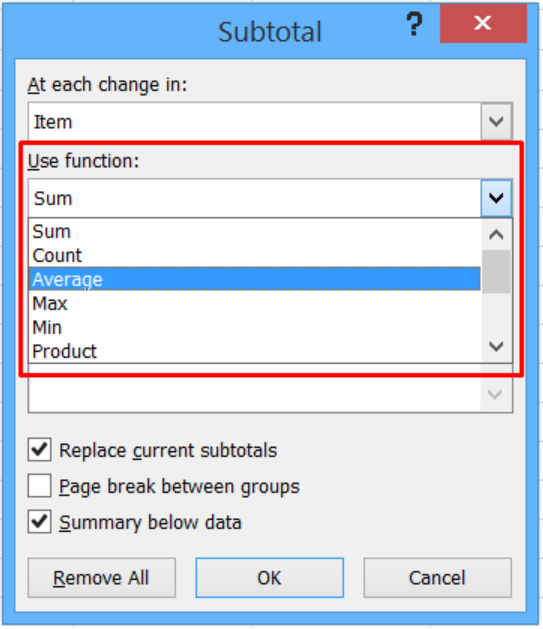Figure 5.  Subtotal functions in Excel

For this example, enter the following values in the textboxes:

At each change in:   Item

Use function: Sum

We choose Sum because it is the function that we want for the calculation of our subtotals. We choose Sales because it contains the values we want to subtotal.  In summary, we want to insert subtotals that show the sum of sales for every item in our list.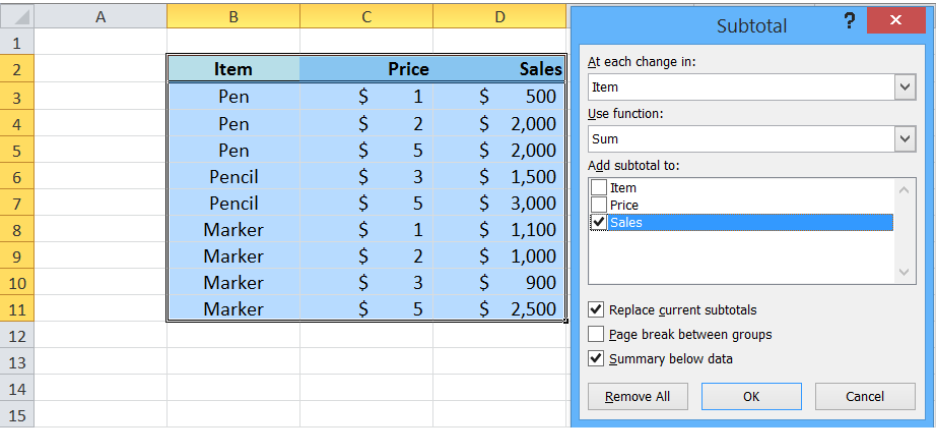Figure 6.  Insert subtotals using the SUM function

The resulting table below shows that we have successfully inserted subtotals in Excel, for Pen, Pencil and Marker.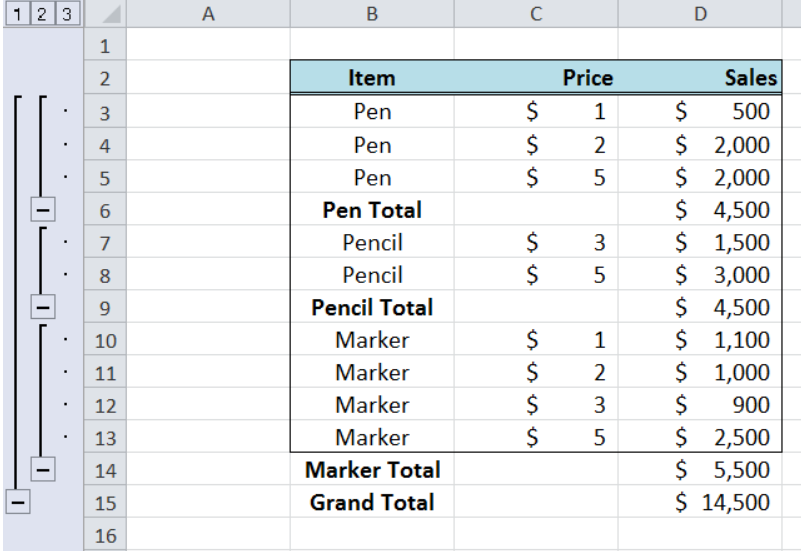Figure 7.  Output:  Insert subtotals in Excel

## Instant Connection to an Expert through our Excelchat Service

Most of the time, the problem you will need to solve will be more complex than a simple application of a formula or function. If you want to save hours of research and frustration, try our live Excelchat service! Our Excel Experts are available 24/7 to answer any Excel question you may have. We guarantee a connection within 30 seconds and a customized solution within 20 minutes.